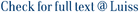We compare estimators of the integral of a monotone function f that can be observed only at a sample of points in its domain, possibly with error. Most of the standard literature considers sampling designs ordered by refinements and compares them in terms of mean square error or, as in Goldstein et al. (2011), the stronger convex order. In this paper we compare sampling designs in the convex order without using partition refinements. Instead we order two sampling designs based on partitions of the sample space, where a fixed number of points is allocated at random to each partition element. We show that if the two random vectors whose components correspond to the number allocated to each partition element are ordered by stochastic majorization, then the corresponding estimators are likewise convexly ordered. If the function f is not monotone, then we show that the convex order comparison does not hold in general, but a weaker variance comparison does.

Stochastic comparisons of symmetric sampling designs / L., Goldstein; Rinott, Joseph; Scarsini, Marco. - In: METHODOLOGY AND COMPUTING IN APPLIED PROBABILITY. - ISSN 1387-5841. - 14:3(2012), pp. 407-420. [10.1007/s11009-011-9213-3]

### Stochastic comparisons of symmetric sampling designs

#### Abstract

We compare estimators of the integral of a monotone function f that can be observed only at a sample of points in its domain, possibly with error. Most of the standard literature considers sampling designs ordered by refinements and compares them in terms of mean square error or, as in Goldstein et al. (2011), the stronger convex order. In this paper we compare sampling designs in the convex order without using partition refinements. Instead we order two sampling designs based on partitions of the sample space, where a fixed number of points is allocated at random to each partition element. We show that if the two random vectors whose components correspond to the number allocated to each partition element are ordered by stochastic majorization, then the corresponding estimators are likewise convexly ordered. If the function f is not monotone, then we show that the convex order comparison does not hold in general, but a weaker variance comparison does.
##### Scheda breve Scheda completa Scheda completa (DC)2012
Convex order; Stochastic majorization; Stratified sampling; Exchangeable partitions of integers
Stochastic comparisons of symmetric sampling designs / L., Goldstein; Rinott, Joseph; Scarsini, Marco. - In: METHODOLOGY AND COMPUTING IN APPLIED PROBABILITY. - ISSN 1387-5841. - 14:3(2012), pp. 407-420. [10.1007/s11009-011-9213-3]
File in questo prodotto:
File
MCAP2012GRS.pdf

Solo gestori archivio

Tipologia: Versione dell'editore
Licenza: DRM (Digital rights management) non definiti
Dimensione 388.81 kB
Utilizza questo identificativo per citare o creare un link a questo documento: `https://hdl.handle.net/11385/17839`
•1
•1